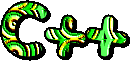## Monday, 17 December 2012

### CBSE Class 11 - C++ Snippets (Part-6)

Graphics Support in TCc++ snippets
Turbo C++ has support for various DOS mode graphics (CGA, VGA, SVGA etc.).

Various graphics functions are supported in GRAPHICS.LIB. You need to include <graphics.h> file in your code.
Following are essential functions to be called to invoke graphics mode:

initgraph( ):  initializes the application to work in graphics mode. It takes three arguments, DETECT, graphics mode and path of *.BGI files.

closegraph( ): closes the graphics mode and switches the application back to test mode.

It supports various graphics functions (e.g. circle, setlinestyle, drawpoly, arc, ellipse...) to draw various graphics primitives. You can fill the graphics object with different colour values and can control the color palette.

Q1:Write a sample program to show graphics mode working of TC++

```/* Simple example to draw circle */
#include <graphics.h>
#include <conio.h>
#include <iostream.h>
#include <stdlib.h>

void main()
{
int gd=DETECT,gm;
initgraph(&gd, &gm, "c:/tc/bgi ");    // initialize graphics mode.
/* read result of initialization */
int errorcode = graphresult();
if (errorcode != grOk)  /* an error occurred */
{
cout << "Graphics error:" <<  grapherrormsg(errorcode) << "\n";
cout << "Press any key to exit.";
getch();
exit(1);             /* return with error code */
}
// randlomly draw 25 circles
int cx, cy, cr, cf, dr, clr;
for (int i = 0; i < 25; ++i)
{
cx = 80 + random(600);    // center of circle (x-point)
cy = 80 + random(280);   // center of circle (y-point)
cr = 20 + random(60);     // inner radius
//cf = 1 +  random(10);     // color fill value
dr = 3 +  random(20);      // outer radius increment
clr = 1 + random(7);       // random color
circle(cx, cy, cr+dr);     // draw outer circle
circle(cx, cy, cr);        // draw inner circle
//floodfill(cx, cy, clr);
setfillstyle(1 + random(10), clr);
floodfill((cx+cr+2), cy, WHITE);
}
//circle(330,180,100);
getch();
closegraph();
restorecrtmode();            // restore text mode
}
```